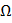# A series AC circuit containing an inductor (20 mH), a capacitor (120and resistor (60) is driven by an AC source of 24V/50Hz.  The energy dissipated in the circuit in 60 s is: a. 2.26x103 J b. 3.39x103J c. 5.65x102J d. 5.17x102J

## Question ID - 50147 :- A series AC circuit containing an inductor (20 mH), a capacitor (120and resistor (60) is driven by an AC source of 24V/50Hz.  The energy dissipated in the circuit in 60 s is: a. 2.26x103 J b. 3.39x103J c. 5.65x102J d. 5.17x102J

3537

R=60f=50Hz,Xc ==Xc=26.52XL==100Xc=XL=20.24=z = 20cos=Pavg =VI CosI==Cos=8.64  watt

Q = P.t = 8.64Next Question :
 The dimensions of the field shown in the given figure areAC = 150 m, AH = 120 m, AG = 80 m, AF = 50 m, EF = 30 m, GB = 50 m, HD = 20 m. The area of this field is (a) 6500 cm2 (b) 6550 cm2 (c) 6600 cm2 (d) 6650 cm2